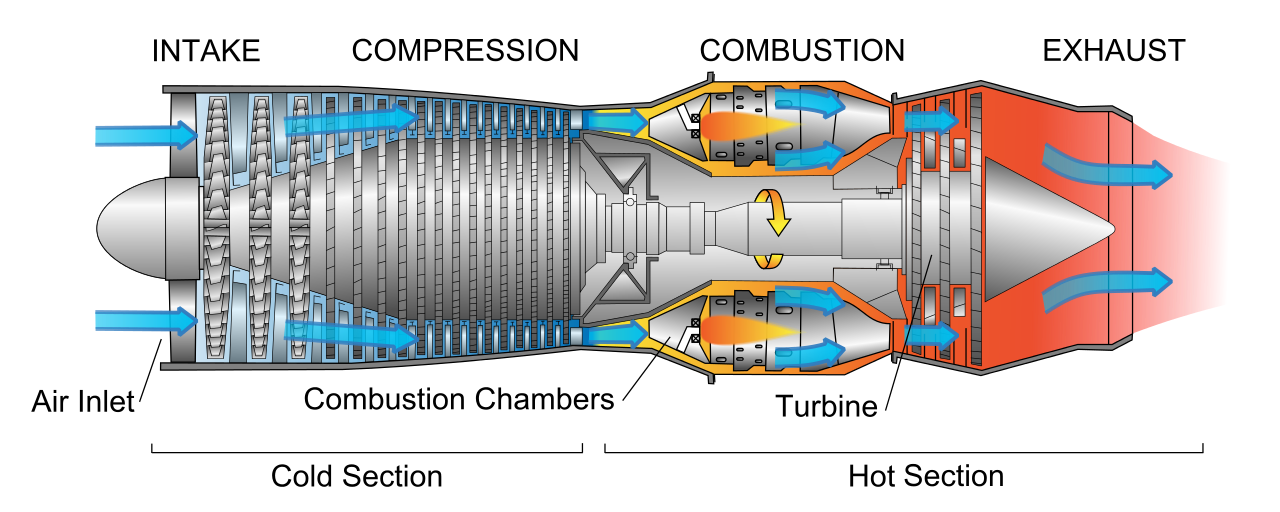#### Problem Statement

An aluminum 5052 jet engine turbine shaft is employed and welded using FSW aluminum. The creep material constants for Al 5052 at the operating temperature isand n = 9.1 and the FSW Al constants are B =and n = 7.627. At what creep stress will the two strain rates be the same? Hint: Set the Strain Rate equations for each material equal to each other.

Strain Rate Equation: